Question

# Using Python Version 1. Write a program that uses a "while" loop to print the first...

Using Python

Version 1. Write a program that uses a "while" loop to print the first 10 positive integers and to compute their sum. Print the sum after it is computed. Do the same with a "for" loop.

Version 2. Write a program to approximate the square root of a number. Recall that the square root of a number x is a number r such that r*r = x. Newton discovered that if one initial estimate of r is z then a better estimate is obtained by taking the average of z and x/z. The estimate can be improved by using this rule again and again until a satisfactory value is obtained (for example, when the difference between x and z*z becomes less than 0.000001 ).

z = 1.0 , then update z inside a while loop using the computation

z = ( z + x/z ) /2

until the difference (x - z**2) has an absolute value less than a given tolerance.

Let tolerance = 0.000001. Print to the screen the value found and compare it with the one returned by math.sqrt(x), that is, the square root function from the math module.

Note on Newton's method: If you have taken calculus, this is using Newton's method to find an approximation of the zeros for a function of the type f(z) = x - z*z, where x is fixed. Newton's method consists in following the equation of the tangent line at the current estimate and using the intersection with the x-axis as an improvement of the estimate. The derivative f'(z) = - 2z gives the slope of the tangent at the current z. Replacing f(z_new) by 0 we get the equation of the tangent line of the form

f(z_old) - 0 = f'(z_old) (z_old - z_new).

z_new = z_old - f(z_old)/f'(z_old)

or for the computer code the update is just

z = z - f(z)/ f'(z)

for the specific f(z) = x - z*z, we have z - f(z)/ f'(z) = (z -( x - z*z)/(-2z)) = z/2 + x/(2z)

which leads to the update statement in the form

z = ( z + x/z ) /2 ;

#VERSION 1
i = 1
sum=0
#while loop
while i <= 10:
print(i) #printing new number on each line
sum += i
i+=1

print("Sum of first 10 positive integers is= "+str(sum))

#for loop

i=1
sum=0
for i in range(1,11):
print(i)
sum+=i
i+=1
print("Sum of first 10 positive integers is= "+str(sum))

#VERSION 2

import math #importing the math lib to use math.sqrt()

x=float(input("Enter x ")) #taking input
z=1.0 #starting z with 1.0 acc. to question
tolerance = 0.000001 #fixing tolerance

while abs(x-z**2)>=tolerance: #looping till the difference doesn't become less than tolerance
z = ( z + x/z ) /2

print("The value computed by our method is           "+str(z))
print("The value computed by math.sqrt() function is "+str(math.sqrt(x)))

#### Earn Coins

Coins can be redeemed for fabulous gifts.

Similar Homework Help Questions
• ### clearvars close all clc tol = 0.0001; % this is the tolerance for root identification xold...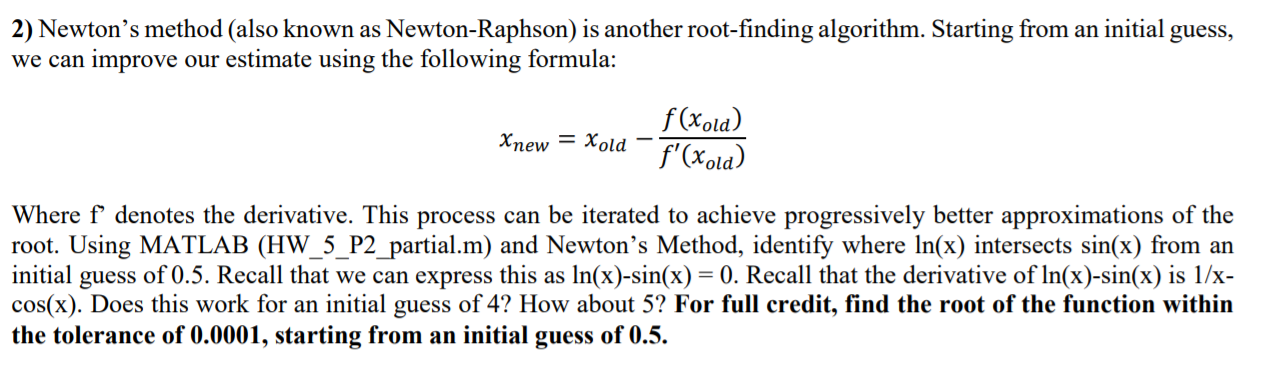clearvars close all clc tol = 0.0001; % this is the tolerance for root identification xold = 0.5; % this is the initial guess test = 1; % this simply ensures we have a test value to enter the loop below. %If we don't preallocate this, MATLAB will error when it trys to start the %while loop below k = 1; %this is the iteration counter. Similar to "test" we need to preallocate it %to allow the while loop to...

• ### Using newton's method calculate to the first 3 iterations. DO NOT WORRY ABOUT THE CODING OR ANYTHING. IHAVE ALRE...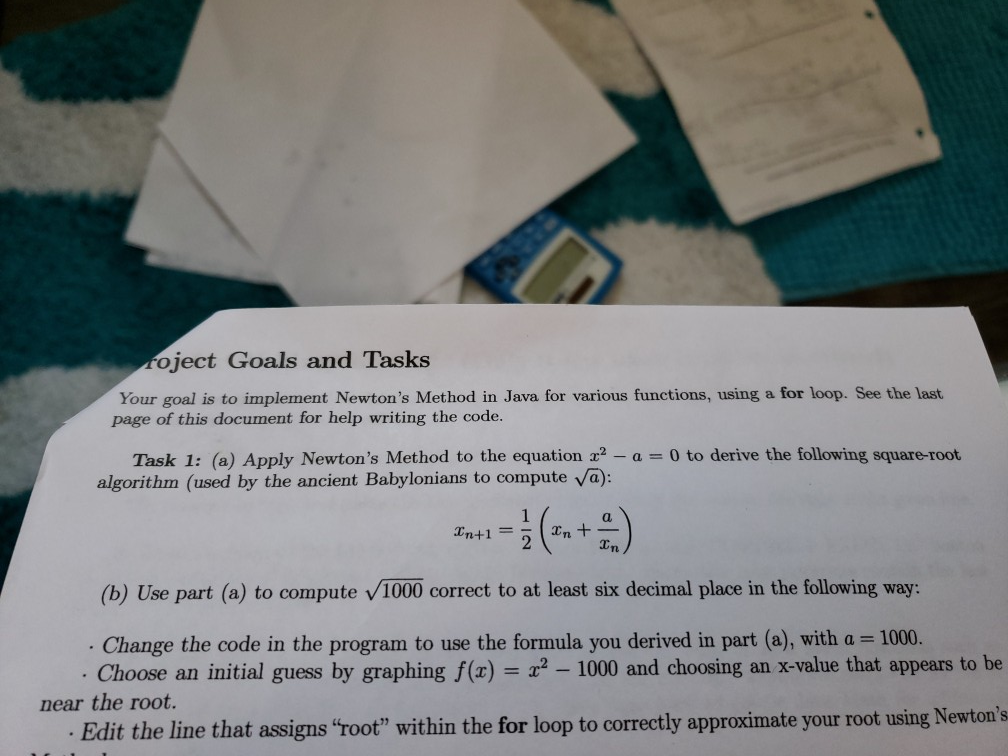Using newton's method calculate to the first 3 iterations. DO NOT WORRY ABOUT THE CODING OR ANYTHING. IHAVE ALREADY COMPLETED THAT. ONLY HAND WRITTEN CALCULATIONS. Foject Goals and Tasks Your goal is to implement Newton's Method in Java for various functions, using a for loop. See the last page of this document for help writing the code. Task 1: (a) Apply Newton's Method to the equation x2 - a = 0 to derive the following square-root algorithm (used by the...

• ### Write a python program that uses a while loop to print numbers 100,98,96... all the way...

Write a python program that uses a while loop to print numbers 100,98,96... all the way to 0. each number should be printed on a seperate line

• ### Please write in Language c using only the files stdio.h and math.h Suppose you wish to...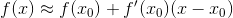Please write in Language c using only the files stdio.h and math.h Suppose you wish to find the root r of a function f(x), that is, the value r where f(r)=0. One method is to make an initial guess, x0, compute the line tangent to f at x0, and find where the tangent line intercepts the x-axis, x1. Then, use x1 as the second guess and repeat this procedure n times until f(xn) approximately equals 0 and report xn as...

• ### Write a program that prints the following console output using the while-loop and for-loop D not...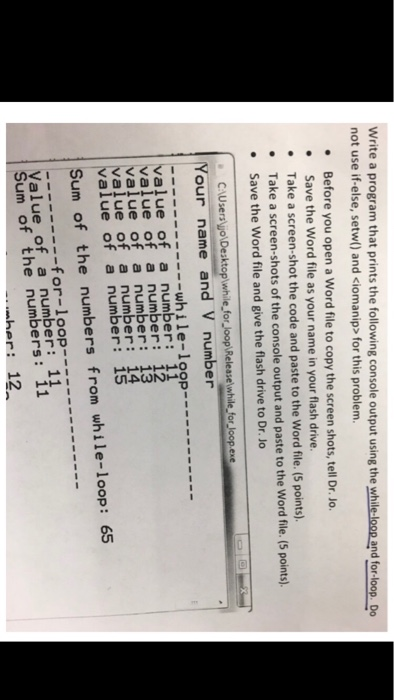Write a program that prints the following console output using the while-loop and for-loop D not use if-else, setw) and ciomanip> for this problem. .Before you open a Word file to copy the screen shots, tell Dr. Jo. Save the Word file as your name in your flash drive. .Take a screen-shot the code and paste to the Word file. (5 points). Take a screen-shots of the console output and paste to the Word file. (5 points) Save the Word...

• ### Step 1. Write a program in assembly language (using macros) to print out the following messages...

Step 1. Write a program in assembly language (using macros) to print out the following messages on the screen [20 marks]: Hello, programmers! Welcome to the world of, Linux assembly programming! Step 2. Write a C function to passed two numbers to the function and calculate the sum of all the numbers between them (both inclusive). [50 marks] a. Make a version with for loop b. Make a version with while loop c. Make a version with do.. while loop...

• ### Please write a java program that inputs positive numbers using a while loop until a negative...

Please write a java program that inputs positive numbers using a while loop until a negative number is input and finds the sum of the numbers input

• ### 1. Determine the root of function f(x)= x+2x-2r-1 by using Newton's method with x=0.8 and error,...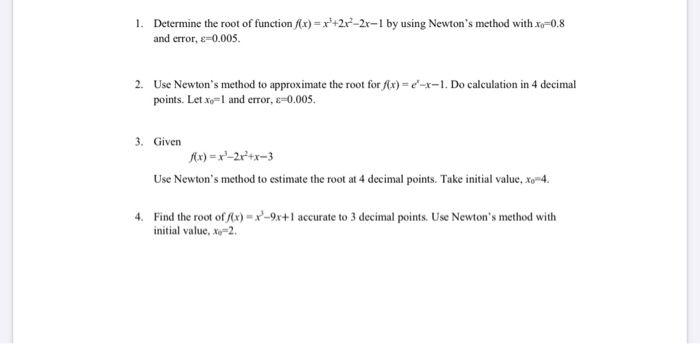1. Determine the root of function f(x)= x+2x-2r-1 by using Newton's method with x=0.8 and error, e=0.005. 2. Use Newton's method to approximate the root for f(x) = -x-1. Do calculation in 4 decimal points. Letx=1 and error, E=0.005. 3. Given 7x)=x-2x2+x-3 Use Newton's method to estimate the root at 4 decimal points. Take initial value, Xo4. 4. Find the root of f(x)=x2-9x+1 accurate to 3 decimal points. Use Newton's method with initial value, X=2

• ### Write a Python program to print all Perfect numbers between 1 to n. (Use any loop...

Write a Python program to print all Perfect numbers between 1 to n. (Use any loop you want) Perfect number is a positive integer which is equal to the sum of its proper positive divisors. Proper divisors of 6 are 1, 2, 3. Sum of its proper divisors = 1 + 2 + 3 = 6. Hence 6 is a perfect number. *** proper divisor means the remainder will be 0 when divided by that number. Sample Input: n =...

• ### Part 1: Using Idle Write a Python Script that Does the Following 1. At the top...

Part 1: Using Idle Write a Python Script that Does the Following 1. At the top of your program, import the math library as in from math import * to make the functions in the math module available. Create a variable and assign into it a constant positive integer number of your choice. The number should be at most 10. 1 Suppose we call this variable x for this writeup document Try something like x = 4 2. Create another...